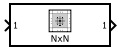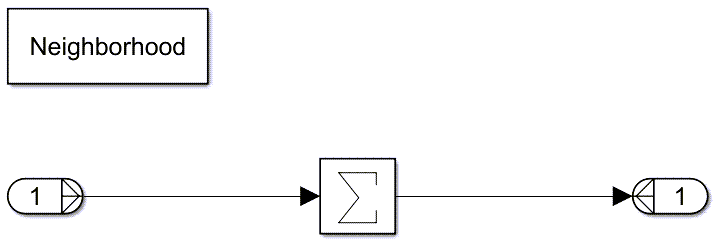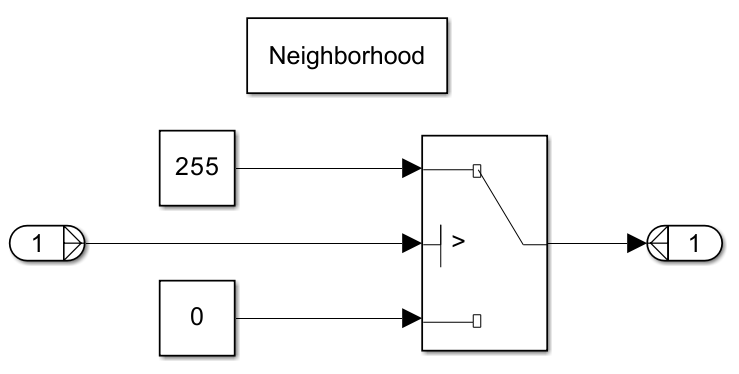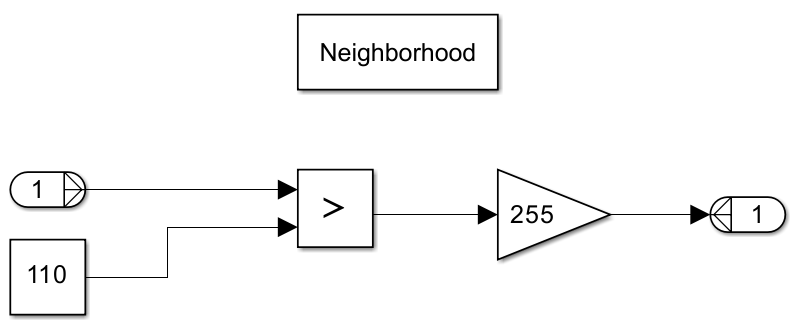# Neighborhood Processing Subsystem

Create algorithm that follows the neighborhood pattern

• Library:

•## Description

The Neighborhood Processing Subsystem block is a Subsystem block preconfigured as a starting point for creating a subsystem that follows the neighborhood pattern. At each simulation time step, the subsystem iterates over each element of an input array. For each element, the subsystem processes a neighborhood of values surrounding that element. Use the subsystem for image processing. Certain image processing algorithms involve processing images in sections rather than processing the entire image at once.

### Configuring Subsystem

The Neighborhood Processing Subsystem contains a Neighborhood block that acts as a control block for the subsystem. Specify the parameters of the Neighborhood block to configure the behavior of the subsystem.

The Neighborhood size parameter controls the dimensions of the neighborhood. For elements near the edges of the input matrix, the neighborhood can extend beyond the input matrix. The Padding option parameter controls how the subsystem treats values from outside the input matrix. You can configure the subsystem to pad these values with a constant or by reusing values from inside the matrix. If you use the `Constant` padding option, use the Padding constant parameter to set the constant value.

The Output size parameter controls the size of the output matrix. The `Same` value configures the output matrix to be the same size as the input matrix. The `Full` value produces a larger output matrix by calculating values for elements outside the input matrix whose neighborhoods include at least one element from the input matrix. The `Valid` value creates a smaller output matrix by calculating values for only the elements whose neighborhoods do not extend beyond the input matrix. When you use this setting, the Padding constant parameter is disabled.

### Example

Consider this model.In this example, the Neighborhood Processing Subsystem block takes a 5-by-5 matrix and outputs a matrix of the same dimensions by using the `Same` output size option. The subsystem contains these blocks.Inside the Neighborhood Processing Subsystem block, the inport does not receive the full 5-by-5 matrix. Instead, because the Neighborhood size parameter value is `[3 3]`, the inport receives a smaller, 3-by-3 window of elements. The subsystem iterates over the elements of the input matrix, passing the neighborhood of each element to the inport at each iteration.

The model uses the `Constant` padding option with a padding value of `0`. For neighborhoods that extend beyond the input matrix, the subsystem treats the external values as `0`.

The outport of the Neighborhood Processing Subsystem block receives a scalar which represents the derived value for the element at the center of the window. In this case, a Sum of Elements block with the Sum over parameter set to `All dimensions` produces a scalar for each window, representing the sum of the values in that window.

Run the model to populate the Display block.Each value of the output matrix is the sum of the corresponding element and its eight direct neighbors in the input matrix. The inner elements are surrounded by values of `1`, and so have the value `9`. The outer elements have lower values because the selected padding method uses the value `0` for elements outside the input matrix.

## Limitations

• The Neighborhood Processing Subsystem block does not support Switch blocks when you use normal simulation mode. To use a Switch block inside a Neighborhood Processing Subsystem block, use the accelerator or rapid accelerator simulation mode. For a workaround in normal simulation mode, see the Tips section.

• The Neighborhood Processing Subsystem block does not support row-major layout.

## Ports

### Input

expand all

Use Inport blocks to get signals from the local environment.

Placing an Inport block in a Neighborhood Processing Subsystem block adds an external input port to the subsystem. The port label matches the name of the Inport block.

Data Types: `single` | `double` | `int8` | `int16` | `int32` | `int64` | `uint8` | `uint16` | `uint32` | `uint64` | `fixed point`

### Output

expand all

Use Outport blocks to send signals to the local environment.

Placing an Outport block in a Neighborhood Processing Subsystem block adds an external output port from the subsystem. The port label matches the name of the Outport block.

Data Types: `single` | `double` | `int8` | `int16` | `int32` | `int64` | `uint8` | `uint16` | `uint32` | `uint64` | `fixed point`

## Block Characteristics

 Data Types `double` | `fixed point` | `integer` | `single` Direct Feedthrough `no` Multidimensional Signals `yes` Variable-Size Signals `no` Zero-Crossing Detection `no`

## Tips

• To improve performance, consider setting the Measure function execution times (Embedded Coder) configuration parameter to ```Coarse (referenced models and subsystems only)``` when you run software-in-the-loop (SIL) or processor-in-the-loop (PIL) profiling on a model that contains a Neighborhood Processing Subsystem block. Neighborhood Processing Subsystem blocks process large amounts of data, which can cause poor performance when you use the `Detailed (all function call sites)` option. For more information, see Create Execution-Time Profile for Generated Code (Embedded Coder).

• Switch blocks are useful in Neighborhood Processing Subsystem blocks for image processing tasks such as thresholding. Thresholding can convert an image to black and white by converting every pixel value to `255` or `0`, depending on whether the input pixel value is greater than a given threshold value. For example, this Neighborhood Processing Subsystem block performs thresholding by using a Switch block with a threshold value of `110`.To perform thresholding in normal simulation mode, consider using a Relational Operator block that uses the `>` operator to recreate the Switch block behavior.The Relational Operator block returns a value of `1` if the input value is greater than the threshold and otherwise returns `0`. By sending this value to a Gain block with a value of `255`, the subsystem returns `255` or `0`.

## Version History

Introduced in R2022b